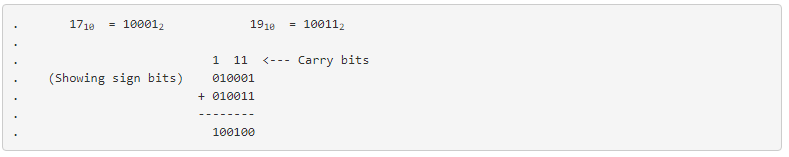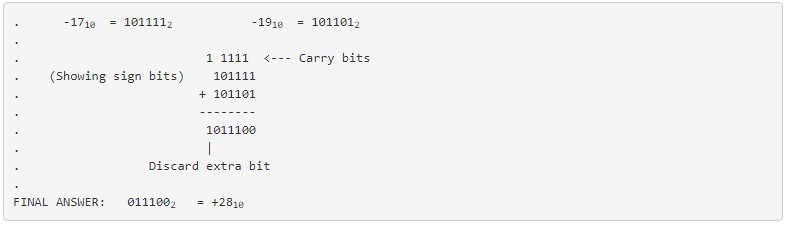2.5: Binary Overflow

One caveat with signed binary numbers is that of overflow, where the answer to an addition or subtraction problem exceeds the magnitude which can be represented with the alloted number of bits. Remember that the place of the sign bit is fixed from the beginning of the problem. With the last example problem, we used five binary bits to represent the magnitude of the number, and the left-most (sixth) bit as the negative-weight, or sign, bit. With five bits to represent magnitude, we have a representation range of 25, or thirty-two integer steps from 0 to maximum. This means that we can represent a number as high as +3110 (0111112), or as low as -3210 (1000002). If we set up an addition problem with two binary numbers, the sixth bit used for sign, and the result either exceeds +3110 or is less than -3210, our answer will be incorrect. Let’s try adding 1710 and 1910 to see how this overflow condition works for excessive positive numbers:The answer (1001002), interpreted with the sixth bit as the -3210 place, is actually equal to -2810, not +3610 as we should get with +1710 and +1910 added together! Obviously, this is not correct. What went wrong? The answer lies in the restrictions of the six-bit number field within which we’re working Since the magnitude of the true and proper sum (3610) exceeds the allowable limit for our designated bit field, we have an overflow error. Simply put, six places doesn’t give enough bits to represent the correct sum, so whatever figure we obtain using the strategy of discarding the left-most “carry” bit will be incorrect. A similar error will occur if we add two negative numbers together to produce a sum that is too low for our six-bit binary field. Let’s try adding -1710and -1910 together to see how this works (or doesn’t work, as the case may be!):The (incorrect) answer is a positive twenty-eight. The fact that the real sum of negative seventeen and negative nineteen was too low to be properly represented with a five bit magnitude field and a sixth sign bit is the root cause of this difficulty. Let’s try these two problems again, except this time using the seventh bit for a sign bit, and allowing the use of 6 bits for representing the magnitude: